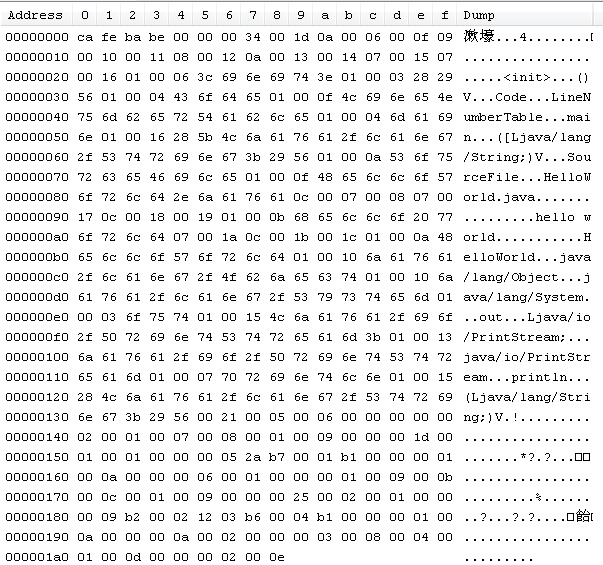# Java常量池理解和经典总结

Java常量池理解和经典总结

1. 什么是常量。

final int i=1024;

2.常量池

 1 2 3 4 5 public class HelloWorld{ public static void main(String args[]){ System.out.println("hello world"); }}

Class文件：1>    魔数

2>    版本号

3>    常量池的入口

4>    常量池

i> 类和接口的全限定名

ii>字段的名称和描述符

iii>方法的名称和描述符

（关于Class文件就先讲到这里，以后有机会再继续）

3.常量池的好处

（1）节省内存空间：常量池中所有相同的字符串常量被合并，只占用一个空间。
（2）节省运行时间：比较字符串时，==比equals()快。对于两个引用变量，只用==判断引用是否相等，也就可以判断实际值是否相等。（摘自博客：http://www.jianshu.com/p/c7f47de2ee80  非常感谢此作者的文章）

4.equals和==的区别

Java中的数据类型分两种：基本数据类型和引用数据类型。

1> 基本数据类型 byte short int long char float double boolean

2> 引用数据类型

  1 2 3 4 5 6 7 8 9 10 11 12 13 14 15 16 17 18 19 20 21 public boolean equals(Object anObject) { if (this == anObject) { return true; } if (anObject instanceof String) { String anotherString = (String)anObject; int n = count; if (n == anotherString.count) { char v1[] = value; char v2[] = anotherString.value; int i = offset; int j = anotherString.offset; while (n-- != 0) { if (v1[i++] != v2[j++]) return false; } return true; } } return false; }

1．对于8种基本数据类型大部分都有自己的封装类，其中Byte,Short,Integer,Long,Character,Boolean都实现了常量池技术。

1>不使用new关键字

Boolean这个就true和false，这个很容易理解。咱们这里可以以Integer为例进行讲解，仔细查看Integer源码你会发现，里面有个静态类IntegerCache。代码如下：

  1 2 3 4 5 6 7 8 9 10 11 12 13 14 15 16 17 18 19 20 21 22 23 24 25 26 private static class IntegerCache { static final int high; static final Integer cache[]; static { final int low = -128; // high value may be configured by property int h = 127; if (integerCacheHighPropValue != null) { // Use Long.decode here to avoid invoking methods that // require Integer's autoboxing cache to be initialized int i = Long.decode(integerCacheHighPropValue).intValue(); i = Math.max(i, 127); // Maximum array size is Integer.MAX_VALUE h = Math.min(i, Integer.MAX_VALUE - -low); } high = h; cache = new Integer[(high - low) + 1]; int j = low; for(int k = 0; k < cache.length; k++) cache[k] = new Integer(j++); } private IntegerCache() {}}

  

  1 2 3 4 5 6 7 public class test { public static void main(String[] args) { Integer i1=10; Integer i2=10; System.out.println(i1==i2); }}

  1 2 3 4 5 6 7 public class test { public static void main(String[] args) { int i1=10; Integer i2=10;// 1.自动装箱 System.out.println(i1==i2);//2.自动拆箱 }}

  1 2 3 4 5 6 7 public static Integer valueOf(int i) { final int offset = 128; if (i >= -128 && i <= 127) { // must cache return IntegerCache.cache[i + offset]; } return new Integer(i); }

2>.使用new关键字，如果使用了new关键字就是在堆内存中开辟了一块内存。每次new一个都是在堆中开辟一块内存，我们可以这样理解，就像我们自己吃苹果，每次都是new一个，我要吃个新的，不要旧的。所以每一个的地址都不一样。

  1 2 3 4 5 6 7 public class test { public static void main(String[] args) { Integer i1=new Integer(10); Integer i2=new Integer(10); System.out.println(i1==i2); }}

3.     没有实现常量池的Float和Double

  1 2 3 4 5 6 7 8 9 10 public class test { public static void main(String[] args) { Float f1=10.0f; Float f2=10.0f; System.out.println(f1==f2); Double d1=12.0; Double d2=12.0; System.out.println(d1==d2); }}

false

false

  1 2 3 4 5 6 7 8 9 10 11 12 13 14 15 16 17 public class test { public static void main(String[] args) { Integer i1=10; Integer i2=10; Integer i3=20; Integer i11=new Integer(10); Integer i22=new Integer(10); Integer i33=new Integer(20); System.out.println(i1==i2); System.out.println(i1==i11); System.out.println(i11==i22); System.out.println(i3==(i1+i2)); System.out.println(i3==(i11+i22)); System.out.println(i33==(i1+i2)); System.out.println(i33==(i11+i22)); }}

true

false

false

true

true

true

true

1> 没有使用new关键字

  1 2 3 4 5 6 7 8 9 10 11 12 public class test { public static void main(String[] args) { String s1="abc1";//此处是数字1 String s2="abc"+1; System.out.println(s1==s2);// 第一次比较 String s3="ab"; String s4="c"; String s5="abc"; String s6=s3+s4; System.out.println(s5==s6);// 第二次比较 }}

true

false

  1 2 3 4 public String toString() { // Create a copy, don't share the array return new String(value, 0, count);}

  1 2 3 4 5 6 7 8 9 public class test { public static final String s1="abc"; public static final String s2="def"; public static void main(String[] args) { String s3=s1+s2; String s4="abcdef"; System.out.println(s3==s4); }}

true

  1 2 3 4 5 6 7 8 9 10 11 12 13 public class test { public static final String s1; public static final String s2; static{ s1="abc"; s2="def"; } public static void main(String[] args) { String s3=s1+s2; String s4="abcdef"; System.out.println(s3==s4); }}

false

2> 使用new关键字

  1 2 3 4 5 6 7 8 public class test { public static void main(String[] args) { String s1=new String("abc"); String s2=new String("abc"); System.out.println(s1==s2); }}

false

*这里还有个重点就是String s1=newString("abc");到底创建了几个对象呢？

i> 类加载时，对于一个类，类加载只会进行一次。此类进行加载时，会把字符串abc放进全局的常量池中，进行保存。

ii> 运行时，当你运行程序的时候，常量池中存在字符串abc,于是把字面量abc拿进heap中，使它的引用交给s1。

3> 动态添加

  1 2 3 4 5 6 7 8 public class test { public static void main(String[] args) { String s1=new String("abc"); String s2=s1.intern(); String s3="abc"; System.out.println(s2==s3); }}

true

 1 2 3 4 5 6 7 8 9 10 11 12 13 14 15 16 17 18 19 20 public class test { private static final String s11="hello"; private static final String s22="world"; public static void main(String[] args) { String s1="hello"; String s2="world"; String s3="helloworld"; String s4=s11+s22; String s5=s1+"world"; String s6=s5.intern(); String s7="hello"+new String("world"); System.out.println(s3==s4); System.out.println(s3==s5); System.out.println(s4==s5); System.out.println(s4==s6); System.out.println(s3==s7); System.out.println(s4==s7); System.out.println(s5==s7); }}

true

false

false

true

false

false

false